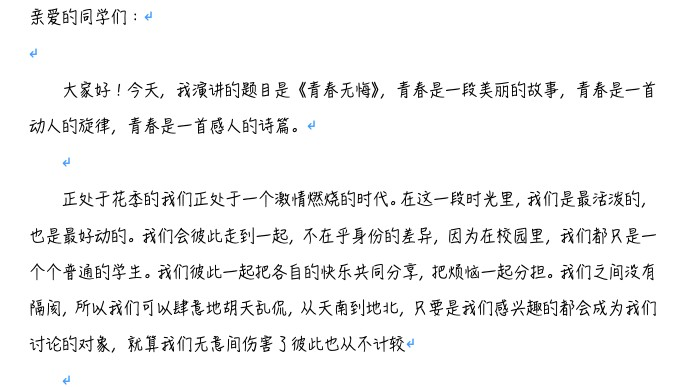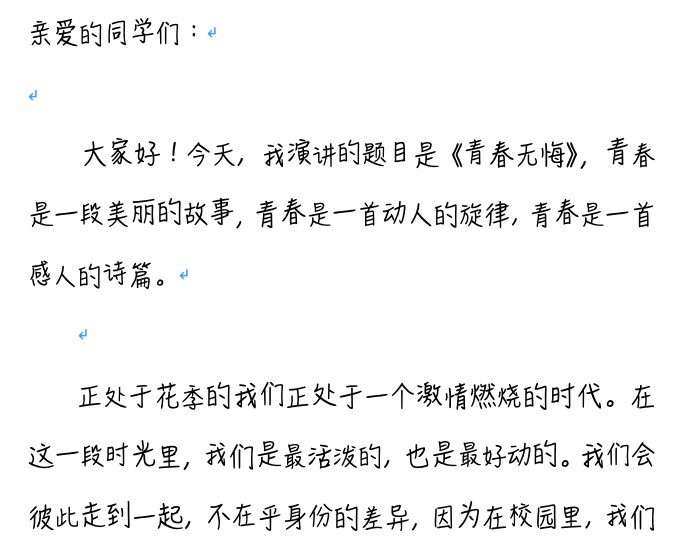#### 利用word的宏命令，将手写效果做得更逼真！ word原创视频教程

9月前 1264 下载全文```Sub 随机字体大小()
'
' 随机字体大小 宏
'
'
Dim R_Character As Range
Dim FontSize(5)
'指定五种字号
FontSize(1) = "16"
FontSize(2) = "16.2"
FontSize(3) = "16.5"
FontSize(4) = "17"
FontSize(5) = "17.2"
Dim ParagraphSpace(5)
'指定五种行间距
ParagraphSpace(1) = "12"
ParagraphSpace(2) = "13"
ParagraphSpace(3) = "17"
ParagraphSpace(4) = "9"
ParagraphSpace(5) = "12"
For Each R_Character In ActiveDocument.Characters
VBA.Randomize
'字号在5种指定大小中随机选取
R_Character.Font.Size = FontSize(Int(VBA.Rnd * 5) + 1)
'位置在1—3之间随机选取
R_Character.Font.Position = Int(VBA.Rnd * 3) + 1
R_Character.Font.Spacing = 0
Next
Application.ScreenUpdating = True
For Each Cur_Paragraph In ActiveDocument.Paragraphs
'行间距在5个指定值中随机选取
Cur_Paragraph.LineSpacing = ParagraphSpace(Int(VBA.Rnd * 5) + 1)

Next
Application.ScreenUpdating = True

End Sub```